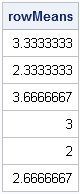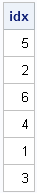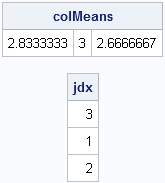In a previous blog post, I showed how to use the SAS/IML SORT and SORTNDX subroutines to sort rows of a matrix according to the values of one or more columns. There is another common situation in which you might need to sort a matrix: you compute a statistic for each row and you want to order the rows according to the value of that statistic.

For example, suppose that each row of the matrix represent a US state and the columns represent data about crimes. For each state (row), you can compute a measure of the severity of crime in the state. You might want to reorder the rows so that low-crime states are listed first and high-crime states are listed last.

The technique that I describe in this article is independent of the size of the matrix. Consequently, I illustrate the technique by using a small 6x3 matrix. The following SAS/IML statements define the matrix and use the mean subscript reduction operator (:) to compute the mean of each row:

```proc iml; x = {5 1 4, 1 5 1, 4 3 4, 2 4 3, 2 3 1, 3 2 3};   /** in general, compute ANY statistic for rows **/ rowMeans = x[,:]; print rowMeans;```The printed output shows the mean for each row. You can use the SORTNDX subroutine to obtain the vector (idx) that sorts the means. If you use that vector as a row subscript for the x matrix, the resulting matrix is sorted according to the row means, as shown in the following statements:

```/** get row numbers that sort the matrix **/ call sortndx(idx, rowMeans, 1); print idx;   /** sort matrix by row statistics **/ y = x[idx, ];```Why does this work? The idx vector indicates that row 5 is the row that has the smallest mean, row 2 is the row that has the second smallest mean, and so on, down to row 3, which is the row that has the largest mean. Consequently, the expression x[idx, ] sorts the rows of x according to their mean values.

Although this example uses the mean of the rows, it is clear that you can reorder the rows according to the values of any statistic.

### Reordering Columns of a Matrix

The technique also applies to reordering columns of a matrix. For example, suppose that you compute the means of each column of x. The following SAS/IML statements reorder the columns so that the column that has the smallest mean is first, and the column that has the largest mean is last:

```/** compute mean for each column **/ colMeans = x[:,]; print colMeans;   /** get col numbers that sort the variables **/ call sortndx(jdx, T(colMeans), 1); /** note T=transpose **/ print jdx;   /** sort matrix by col statistics **/ z = x[, jdx];```Notice that the vector jdx is used as a column index for the x matrix. Except for that difference, these statements are essentially the same as the statements in the previous section.

Share